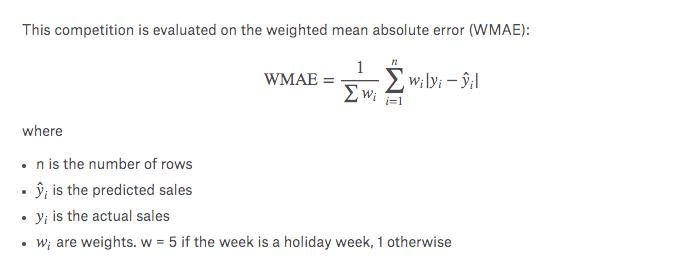# How to calculate Weighted Mean Absolute Error (WMAE)?

Hey.

I’m stuck on this for a while.
Please could you help in calculating the WMAE.

From Kaggle Walmart COmpetition Page:Pseudo code:

``````def exp_wmae(y_pred, targ):
if holiday_week:
return (targ - y_pred)*5
else
return (targ - y_pred)
``````

I want the WMAE error to be displayed each time I call the fit function.
I have a column holiday_week which checks for holiday, but wondering how do I access this column holiday_week while the model is training?

Just having trouble understanding this.
I looked at Rossmann code. For Rossman it was RMSE and it was passed via metrics.

``````def inv_y(a): return np.exp(a)

def exp_rmspe(y_pred, targ):
targ = inv_y(targ)
pct_var = (targ - inv_y(y_pred))/targ
return math.sqrt((pct_var**2).mean())

max_log_y = np.max(yl)
y_range = (0, max_log_y*1.2)
``````
``````m.fit(lr, 3, metrics=[exp_rmspe], cycle_len=1)
``````

There was no weighted mean in exp_rmspe. But here we need to check if each prediction is a holiday_week and accordingly add a weight to the formula.

My question is how do I check/access this holiday_week inside each prediction? Not sure if I am doing this correctly, as I am not able to understand how to do this at all. Also, not sure what y_range is doing in the Rossmann code.

Thanks for your helpArjun

not completely sure but we also need to have the count of
`holiday_week: or not`
and divide by

``````5*count_holiday_week + 1*(len(week)-count_holiday_week)
``````

as we have a `sigma w` in the denominator also

Have a look at the excel’s pic by (sir) Anandsaha below to make things crystal clear…
(Forgot that we have excel also).

2 Likes

If I am following the formula right, this is the calculation I get on dummy data:

1 Like

Thanks @ecdrid and @anandsaha … Am not sure of the formula muself, but good idea to check via excel and see. Will do that.

I’m lost on how to get holiday_week from y_pred inside the function? The actual code while fitting the model. Or do I make another df containing only weights and pass that along into exp_wmae function?

Can you add a screenshot of `df.head()` so that anyone from the forum will help to create the custom function… otherwise we couldn’t connect the dots…

Sure… This is df that I use while creating the model using

``````md = ColumnarModelData.from_data_frame(PATH, val_idx, df, yl, cat_flds=cat_vars, bs=128,
test_df=df_test)
``````

IsHoliday is the column which says if that week is a holiday or not.

Just lost on how to access this column inside y_pred, so that the `exp_wmae` function can multiply by 5 if it is a holiday week.

Thanks.

I know this is an old thread, but I found it useful, so in case anyone needs a little more help, I have written the code for the WMAE based on the excel document posted by @anandsaha

``````def wmae_new(y_pred, targ, holiday_week):
sumOfWeights = 0
sumofCol_B_X_Col_E = 0

for i in range(0, len(y_pred)):
weight = 0
if holiday_week[i]:
weight = 5
else:
weight = 1

Col_B_X_Col_E = abs(targ[i] - y_pred[i])*weight
sumOfWeights += weight
sumofCol_B_X_Col_E += Col_B_X_Col_E
WMAE = sumofCol_B_X_Col_E/sumOfWeights
return WMAE``````

Hi,
after reading different posts and docs I finally was able to implement the Weighted MAE in a callback class that I can pass to the learner and calculate the metric at training time!!!
My version doesn’t use any passed parameters but gets the needed information directly from the training input data.
Here the code:

``````class Weighted_Mae(LearnerCallback):
_order=-20 # Needs to run before the recorder
def __init__(self, learn):
super().__init__(learn)
self.w_sum = 0
self.numerator_sum = 0
self.output_list = []
self.target_list = []
self.w_mae = 0
self.input_list = []

def on_epoch_begin(self, **kwargs):
self.w_sum = 0
self.numerator_sum = 0
self.output_list = []
self.target_list = []
self.input_list = []
self.w_mae = 0

def on_batch_begin(self, last_input, last_target, **kwargs):
# Here I'm taking only the categorical variables from the input tensor
# last_input = categorical variables
# last_input = continuos variables
self.input_list.append(last_input)

def on_batch_end(self, last_output, last_target, **kwargs):
self.output_list.append(last_output.flatten())
self.target_list.append(last_target)

def on_train_begin(self, **kwargs):

def on_epoch_end(self, last_metrics, **kwargs):
self.output_list = torch.cat(self.output_list)
self.target_list = torch.cat(self.target_list)
self.input_list = torch.cat(self.input_list)
for pred,targ,inp in zip(self.output_list, self.target_list, self.input_list):
w = 1
if inp: # the third categorical variable is IsHoliday
w = 5
self.w_sum += w
self.numerator_sum += w*(abs(targ-pred))
self.w_mae = self.numerator_sum/self.w_sum
``````learn = tabular_learner(data, layers=[400,200,100], ps=[0.001,0.01, 0.1], y_range=y_range, emb_drop=0.04,
Maybe this could help somebody